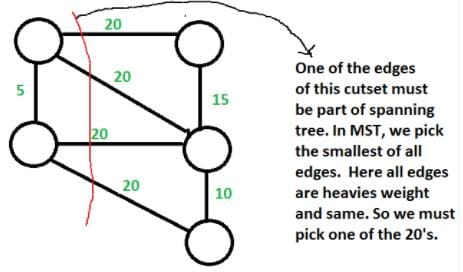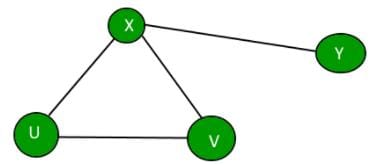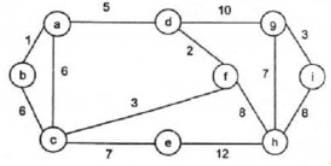Test: Graph - 1

# Test: Graph - 1

Test Description

## 15 Questions MCQ Test Programming and Data Structures | Test: Graph - 1

Test: Graph - 1 for Computer Science Engineering (CSE) 2023 is part of Programming and Data Structures preparation. The Test: Graph - 1 questions and answers have been prepared according to the Computer Science Engineering (CSE) exam syllabus.The Test: Graph - 1 MCQs are made for Computer Science Engineering (CSE) 2023 Exam. Find important definitions, questions, notes, meanings, examples, exercises, MCQs and online tests for Test: Graph - 1 below.
Solutions of Test: Graph - 1 questions in English are available as part of our Programming and Data Structures for Computer Science Engineering (CSE) & Test: Graph - 1 solutions in Hindi for Programming and Data Structures course. Download more important topics, notes, lectures and mock test series for Computer Science Engineering (CSE) Exam by signing up for free. Attempt Test: Graph - 1 | 15 questions in 45 minutes | Mock test for Computer Science Engineering (CSE) preparation | Free important questions MCQ to study Programming and Data Structures for Computer Science Engineering (CSE) Exam | Download free PDF with solutions
 1 Crore+ students have signed up on EduRev. Have you?
Test: Graph - 1 - Question 1

### The most efficient algorithm for finding the number of connected components in an undirected graph on n vertices and m edges has time complexity.

Detailed Solution for Test: Graph - 1 - Question 1

Connected components can be found in O(m + n) using Tarjan’s algorithm. Once we have connected components, we can count them.

Test: Graph - 1 - Question 2

### Consider an undirected unweighted graph G. Let a breadth-first traversal of G be done starting from a node r. Let d(r, u) and d(r, v) be the lengths of the shortest paths from r to u and v respectively, in G. lf u is visited before v during the breadth-first traversal, which of the following statements is correct?

Detailed Solution for Test: Graph - 1 - Question 2

d(r, u) and d(r, v) will be equal when u and v are at same level, otherwise d(r, u) will be less than d(r, v)

Test: Graph - 1 - Question 3

### How many undirected graphs (not necessarily connected) can be constructed out of a given set V= {V 1, V 2,…V n} of n vertices ?

Detailed Solution for Test: Graph - 1 - Question 3

In an undirected graph, there can be maximum n(n-1)/2 edges. We can choose to have (or not have) any of the n(n-1)/2 edges. So, total number of undirected graphs with n vertices is 2^(n(n-1)/2).

Test: Graph - 1 - Question 4

Which of the following statements is/are TRUE for an undirected graph?
P: Number of odd degree vertices is even
Q: Sum of degrees of all vertices is even

Detailed Solution for Test: Graph - 1 - Question 4

P is true for undirected graph as adding an edge always increases degree of two vertices by 1.

Q is true: If we consider sum of degrees and subtract all even degrees, we get an even number because every edge increases the sum of degrees by 2. So total number of odd degree vertices must be even.

Test: Graph - 1 - Question 5

Consider an undirected random graph of eight vertices. The probability that there is an edge between a pair of vertices is 1/2. What is the expected number of unordered cycles of length three?

Detailed Solution for Test: Graph - 1 - Question 5

A cycle of length 3 can be formed with 3 vertices. There can be total 8C3 ways to pick 3 vertices from 8. The probability that there is an edge between two vertices is 1/2. So expected number of unordered cycles of length 3 = (8C3)*(1/2)3 = 7

Test: Graph - 1 - Question 6

Given an undirected graph G with V vertices and E edges, the sum of the degrees of all vertices is

Detailed Solution for Test: Graph - 1 - Question 6

Since the given graph is undirected, every edge contributes as 2 to sum of degrees.
So the sum of degrees is 2E.

Test: Graph - 1 - Question 7

What is the maximum number of edges in an acyclic undirected graph with n vertices?

Detailed Solution for Test: Graph - 1 - Question 7

n * (n – 1) / 2 when cyclic. But acyclic graph with the maximum number of edges is actually a spanning tree and therefore, correct answer is n-1 edges.

Test: Graph - 1 - Question 8

Let G be a weighted undirected graph and e be an edge with maximum weight in G. Suppose there is a minimum weight spanning tree in G containing the edge e. Which of the following statements is always TRUE?

Detailed Solution for Test: Graph - 1 - Question 8

Background : Given a connected and undirected graph, a spanning tree of that graph is a subgraph that is a tree and connects all the vertices together.

1. A spanning tree has exactly V – 1 edges.
2. A single graph can have many different spanning trees. A minimum spanning tree (MST) or minimum weight spanning tree for a weighted, connected and undirected graph is a spanning tree with weight less than or equal to the weight of every other spanning tree. The weight of a spanning tree is the sum of weights given to each edge of the spanning tree.
3. There can be more that one possible spanning trees of a graph. For example, the graph in this question has 6 possible spanning trees.
4. MST has lightest edge of every cutset. Remember Prim’s algorithm which basically picks the lightest edge from every cutset.

Choices of this question :
a) There exists a cutset in G having all edges of maximum weight : This is correct. If there is a heaviest edge in MST, then there exist a cut with all edges with weight equal to heavies edge. See point 4 discussed in above background.b) There exists a cycle in G having all edges of maximum weight : Not always TRUE. The cutset of heaviest edge may contain only one edge. In fact there may be overall one edge of heavies weight which is part of MST (Consider a graph with two components which are connected by only one edge and this edge is the heavies)

c) Edge e cannot be contained in a cycle. Not Always True. The curset may form cycle with other edges.

d) All edges in G have the same weight Not always True. As discussed in option b, there can be only one edge of heaviest weight.

Test: Graph - 1 - Question 9

The cyclomatic complexity of the flow graph of a program provides

Detailed Solution for Test: Graph - 1 - Question 9

Option (C) is correct, because the cyclomatic complexity of the flow graph of a program provides an upper bound for the number of tests that must be conducted to ensure that all statements have been executed at least once.

Test: Graph - 1 - Question 10

What is the largest integer m such that every simple connected graph with n vertices and n edges contains at least m different spanning trees?

Detailed Solution for Test: Graph - 1 - Question 10

A graph is connected iff all nodes can be traversed from each node. For a graph with n nodes, there will be n-1 minimum number of edges.
Given that there are n edges, that means a cycle is there in the graph.
The simplex graph with these conditions may be:Now we can make a different spanning tree by removing one edge from the cycle, one at a time.
Minimum cycle length can be 3, So, there must be atleast 3 spanning trees in any such Graph.

Test: Graph - 1 - Question 11

For the undirected, weighted graph given below, which of the following sequences of edges represents a correct execution of Prim’s algorithm to construct a Minimum Span­ning Tree?Detailed Solution for Test: Graph - 1 - Question 11

In prims algorithm we start with any node and keep on exploring minimum cost neighbors of nodes already covered.

Test: Graph - 1 - Question 12

Let G be a simple graph with 20 vertices and 8 components. If we delete a vertex in G, then number of components in G should lie between ____.

Detailed Solution for Test: Graph - 1 - Question 12

Case 1: If the vertex we are deleting from G is an isolated vertex, which is a component by itself, then number of components in G becomes 7.
Case 2: If G is a start Graph, then by deleting the cut vertex of G, we get 19 components.

Hence, number of components in G should lie between 7 and 19.

Test: Graph - 1 - Question 13

Which of the following data structure is useful in traversing a given graph by breadth first search?

Detailed Solution for Test: Graph - 1 - Question 13

BFS performs level-order traversal which can be fairly done using a queue. A queue uses FIFO ordering and the nodes that we enqueue first are explored first maintaining the order of traversal.

Test: Graph - 1 - Question 14

Which kind of traversal order trie gives the lexicographical sorting of the set of the strings?

Detailed Solution for Test: Graph - 1 - Question 14

To print the string in alphabetical order we have to first insert in the trie and then perform preorder traversal to print in alphabetical order.

Test: Graph - 1 - Question 15

You are given a graph containing n vertices and m edges and given that the graph doesn’t contain cycle of odd length. Time Complexity of the best known algorithm to find out whether the graph is bipartite or not is ?

Detailed Solution for Test: Graph - 1 - Question 15

It is by definition that a graph is bipartite iff it does not contain odd length cycles.

## Programming and Data Structures

71 docs|29 tests
 Use Code STAYHOME200 and get INR 200 additional OFF Use Coupon Code
Information about Test: Graph - 1 Page
In this test you can find the Exam questions for Test: Graph - 1 solved & explained in the simplest way possible. Besides giving Questions and answers for Test: Graph - 1, EduRev gives you an ample number of Online tests for practice

71 docs|29 tests